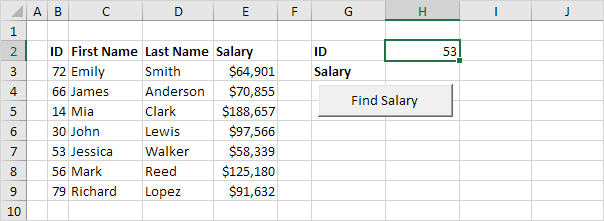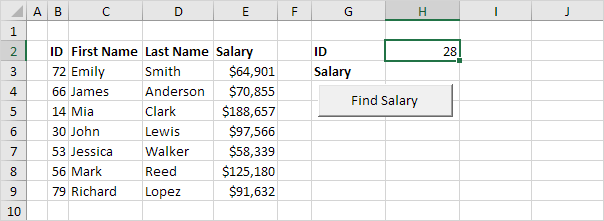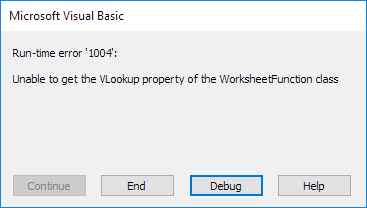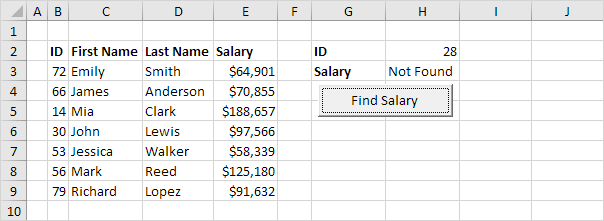# Vlookup

Use the WorksheetFunction property in Excel VBA to access the VLOOKUP function. All you need is a single code line.

Situation:1. Place a command button on your worksheet and add the following code line:

Range("H3").Value = WorksheetFunction.VLookup(Range("H2"), Range("B3:E9"), 4, False)

When you click the command button on the worksheet, Excel VBA uses the VLOOKUP function to lookup the salary of ID 53 and places the result into cell H3.If the VLOOKUP function cannot find a match, Excel VBA returns the "Unable to get the VLookup property of the WorksheetFunction class" error.

2. For example, enter the value 28 into cell H2.Result when you click the command button on the sheet:3. To replace this error with a friendly message, update the code as follows.

On Error GoTo InvalidValue:

Range("H3").Value = WorksheetFunction.VLookup(Range("H2"), Range("B3:E9"), 4, False)

Exit Sub